# base ratio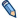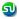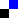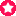## Значение термина base ratio в knolik

base ratio - base ratio
base ratio - In DNA, the amount of adenine (A) and thymine (T) (which are paired, See also: Base pairing) equals the amount of guanine (G) and cytosine (C) (also paired). But the ratio of the amount of A plus T to that of G plus C, which is the base ratio, varies widely.

## Рядом со словом base ratio в knolik

 base pairing В началобуква ""буквосочетание "" basic dyes

Статья про base ratio была прочитана 415 раз

Our friends, knolik encyclopaedia knolik.com